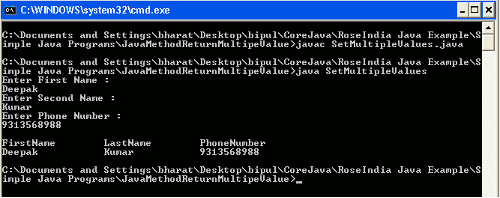Tutorials

# Java Method Return Multiple Values

This example explains you how a multiple values can be return by a method. This example explains you all the steps required in to return multiple values in Java. In this example you will see how we can return multiple values using the return statement.

This example explains you how a multiple values can be return by a method. This example explains you all the steps required in to return multiple values in Java. In this example you will see how we can return multiple values using the return statement.

## Java Method Return Multiple Values

In this section we will learn about how a method can return multiple values in Java.

This example explains you how a multiple values can be return by a method. This example explains you all the steps required in to return multiple values in Java. In this example you will see how we can return multiple values using the return statement.

Example

Here I am giving a simple example into which you will see how a return statement returns the multiple values. In this example I have created a class named GetMultipleValues.java which contains some fields, constructor with fields, and the getter method of the fields. Then I have created another class named SetMultipleValues.java which also contains some fields then created a method named returnValue() into which I have takes the input at command line and set their value into the variables and return these values using return statement then in the main() method I have created an object of SetMultipleValues class and called the returnValue() method and to get these values I have called the getter method of GetMultipleValues class.

Source Code

GetMultipleValues.java

```package roseindia.net.example;

public class GetMultipleValues {

String firstName, lastName;
int phoneNumber;

public GetMultipleValues(String firstName, String lastName,
int phoneNumber) {
this.firstName = firstName;
this.lastName = lastName;
this.phoneNumber = phoneNumber;
}
public String getFirstName() {
return firstName;
}

public String getLastName() {
return lastName;
}

public int getPhoneNumber() {
return phoneNumber;
}

}
```

SetMultipleValues.java

```package roseindia.net.example;

import java.util.Scanner;

public class SetMultipleValues {
String fName, lName;
int phNum;

public GetMultipleValues returnValue()
{
Scanner scan = new Scanner(System.in);
System.out.println("Enter First Name : ");
fName = scan.next();

System.out.println("Enter Second Name : ");
lName = scan.next();

System.out.println("Enter Phone Number : ");
phNum = Integer.parseInt(scan.next());

return new GetMultipleValues(fName,lName,phNum);
}

public static void main(String args[])
{
SetMultipleValues smv = new SetMultipleValues();
GetMultipleValues gmv = smv.returnValue();
System.out.println(gmv.getFirstName()+gmv.getLastName()+gmv.getPhoneNumber());
}

}
```

Output